# C Program to Reverse an Array

In this tutorial, you will learn and get code for reversing an array in C. Reversing an array means

• The first element becomes the last, and the last element becomes the first.
• Similarly, the second element is transformed into the second last, and the second last element is transformed into the second.
• and so on.

For example, if the given array is:

`2 6 4 8`

then the inverse will be

`8 4 6 2`

## Print the reverse of an array in C

Before we change the order of the array, let's make a program that takes 10 array elements and prints them in the reverse order.

To print the array elements in reverse order, start their indexing from last to first. That is, if the user has provided 10 array elements, then the array present at index number 9 will be printed first and the element present at index number 0 will be printed last. In this way, the array in reverse order gets printed as shown in the program given below:

```#include<stdio.h>
#include<conio.h>
int main()
{
int arr, i;
printf("Enter any 10 elements: ");
for(i=0; i<10; i++)
{
scanf("%d", &arr[i]);
}
printf("\nThe array elements in reverse order:\n");
for(i=9; i>=0; i--)
{
if(i==0)
printf("%d", arr[i]);
else
printf("%d, ", arr[i]);
}
getch();
return 0;
}```

The program given above was written in the Code::Blocks IDE; therefore, after a successful build and run, here is its sample run: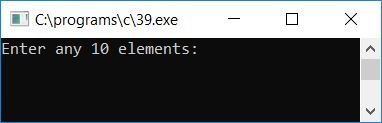Supply any 10 array elements and press the ENTER key to see the given 10 array elements in reverse order, as shown in the second snapshot of the sample run: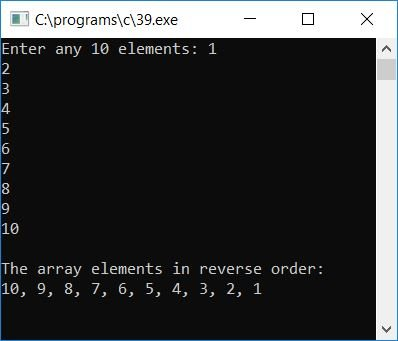## C Reverse an Array

Now let's modify the above program in a way that it will receive the size of an array, say 5. It will then prompt you to enter 5 array elements. After receiving the array elements, this program will reverse the array and then print the array on the output. In the previous program, we have printed the array in reverse order without actually reversing it.

```#include<stdio.h>
#include<conio.h>
int main()
{
int arr, size, i, j, temp;
printf("Enter array size: ");
scanf("%d",&size);
printf("Enter %d array elements: ", size);
for(i=0; i<size; i++)
scanf("%d",&arr[i]);
j=i-1;     // now j will point to the last element
i=0;       // and i will be point to the first element
while(i<j)
{
temp=arr[i];
arr[i]=arr[j];
arr[j]=temp;
i++;
j--;
}
printf("\nReverse of the Array is:\n");
for(i=0; i<size; i++)
printf("%d ",arr[i]);
getch();
return 0;
}```

The program was written under the Code::Blocks IDE, and here is the first snapshot of the sample run: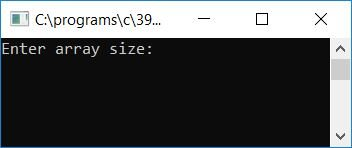Supply the size of the array, say 5, and then enter any 5 array elements, pressing the ENTER key to reverse the array. And print its reverse as shown in the second snapshot of the sample run: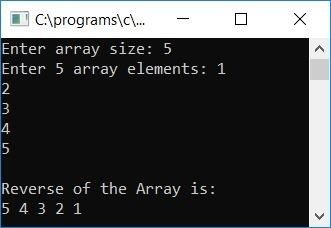#### Program Explained

• Set the size of the receive array to 5, for example.
• Then receive 5 array elements.
• Using a while loop, as the size of the array here is 5, the element present at last, that is, the element present at the 4th index, will be initialized to the 0th index, and the element present at the 0th index will be initialized to the 4th index, and then the 3rd will be initialized to the 1st, and the 1st will be initialized to the 3rd, and so on.
• In this way, we can reverse the array as shown in the above program.
• Finally, after reversing the array, print it.

## C Print the Original and Reverse Arrays

Let's create one more program that will receive the size and elements of an array, and will print the array elements in original and reverse order:

```#include<stdio.h>
#include<conio.h>
int main()
{
int arr, i, limit;
printf("How many elements you want to store inside the array: ");
scanf("%d", &limit);
printf("Enter any %d elements: ", limit);
for(i=0; i<limit; i++)
{
scanf("%d", &arr[i]);
}
printf("\nThe array elements are:\n");
for(i=0; i<limit; i++)
{
if(i==(limit-1))
printf("%d", arr[i]);
else
printf("%d, ", arr[i]);
}
printf("\nNow the array elements in reverse order:\n");
for(i=(limit-1); i>=0; i--)
{
if(i==0)
printf("%d", arr[i]);
else
printf("%d, ", arr[i]);
}
getch();
return 0;
}```

Here is the sample run: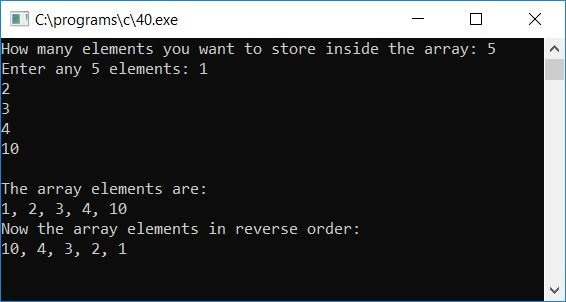#### The same program in different languages

C Quiz

« Previous Program Next Program »